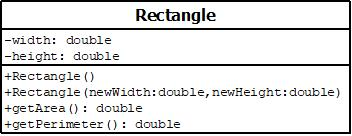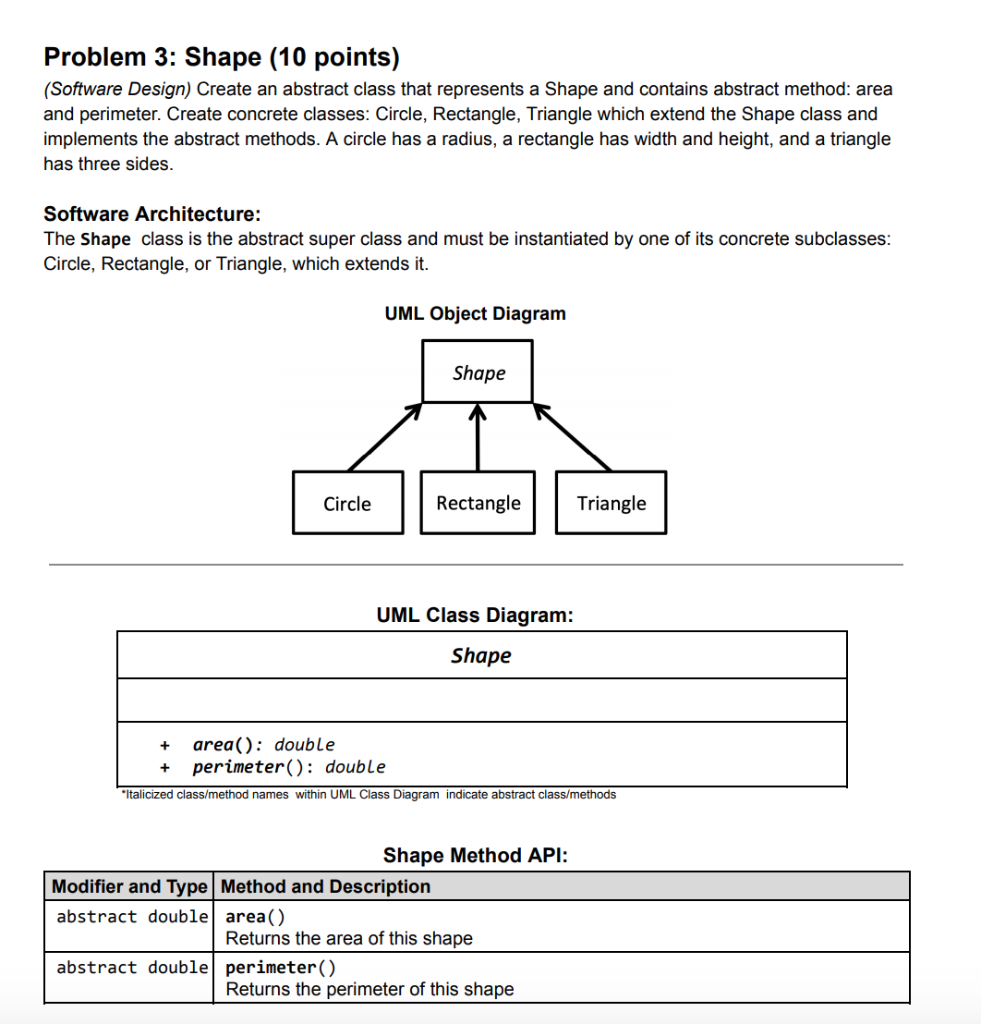# JAVA design a class named Rectangle to represent a rectangle. The class contains: A method named getPerimeter() that returns the perimeter. Two double data fields named width and height that specify...

JAVA

design a class named Rectangle to represent a rectangle. The class contains:

A method named getPerimeter() that returns the perimeter.

• Two double data fields named width and height that specify the width and height of the rectangle. The default values are 1 for both width and height.

• A no-arg constructor that creates a default rectangle.

• A constructor that creates a rectangle with the specified width and height.

• A method named getArea() that returns the area of this rectangle.

• design a class named Rectangle to represent a rectangle. The class contains:

• Two double data fields named width and height that specify the width and height of the rectangle. The default values are 1 for both width and height.

• A no-arg constructor that creates a default rectangle.

• Draw the UML diagram for the class and then implement the class. Write a test program that creates two Rectangle objects-one with width 4 and height 40 and the other with width 3.5 and height 35.9. Display the width, height, area, and perimeter of each rectangle in this order.

• A constructor that creates a rectangle with the specified width and height.

• A method named getArea() that returns the area of this rectangle.

• A method named getPerimeter() that returns the perimeter.

Draw the UML diagram for the class and then implement the class. Write a test program that creates two Rectangle objects-one with width 4 and height 40 and the other with width 3.5 and height 35.9. Display the width, height, area, and perimeter of each rectangle in this order.

public class Exercise09_01
{
public static void main (String[] args)
{
Rectangle rectangle1 = new Rectangle(4,40);

Rectangle rectangle2 = new Rectangle(3.5,3.59);

System.out.println("The details of the first rectangel...");
System.out.println("Width of Rectangle1:" + rectangle1.width);
System.out.println("Height of Rectangle1:" + rectangle1.height);
System.out.println("Area of Rectangle1:" + df.format(rectangle1.getArea()));
System.out.println("Perimeter of Rectangle1:"+ rectangle1.getPerimeter());
System.out.println();

System.out.println("The details of the second rectangel...");
System.out.println("Width of Rectangle2:" + rectangle2.width);
System.out.println("Height of Rectangle2:" + rectangle2.height);
System.out.println("Area of Rectangle2:" + df.format(rectangle2.getArea()));
System.out.println("Perimeter of Rectangle2:"+ rectangle2.getPerimeter());
}
}

public class Rectangle
{
double width = 1.0;
double height = 1.0;
Rectangel() {
}
Rectangel(double newWidth, double newHeight)
{
width = newWidth;
height = newHeight;
}
double getArea()
{
return width * height;
}
double getPerimeter()
{
return 2*(width + height);
}
}

UML Rectangle class digaram-------------------------------*-------------------------------*-------------------------------*
/**Test java program for Rectangle class*/
//Exercise09_01.java
import java.text.DecimalFormat;
public class Exercise09_01
{
public static void main (String[] args)
{
Rectangle rectangle1 = new Rectangle(4,40);
Rectangle rectangle2 = new Rectangle(3.5,3.59);

/**Create an instace of DecimalFormat class*/
DecimalFormat df=new DecimalFormat("#,##.##");

System.out.println("The details of the first rectangel...");
System.out.println("Width of Rectangle1:" + rectangle1.width);
System.out.println("Height of Rectangle1:" + rectangle1.height);
System.out.println("Area of Rectangle1:" + df.format(rectangle1.getArea()));
System.out.println("Perimeter of Rectangle1:"+ rectangle1.getPerimeter());
System.out.println();

System.out.println("The details of the second rectangel...");
System.out.println("Width of Rectangle2:" + rectangle2.width);
System.out.println("Height of Rectangle2:" + rectangle2.height);
System.out.println("Area of Rectangle2:" + df.format(rectangle2.getArea()));
System.out.println("Perimeter of Rectangle2:"+ rectangle2.getPerimeter());
}
}

-------------------------------*-------------------------------*-------------------------------*
//Rectangle.java
public class Rectangle
{
/**Instance variables */
double width ;
double height;
/*Name of the constructor must match with
* the name of the class, Rectangle*/
public Rectangle()
{
width=1;
height=1;
}
/*Name of the constructor must match with
* the name of the class, Rectangle*/
public Rectangle(double newWidth, double newHeight)
{
width = newWidth;
height = newHeight;
}
/**Returns area */
public double getArea()
{
return width * height;
}
/**Returns perimeter */
public double getPerimeter()
{
return 2*(width + height);
}
}/**End of Rectangle class*/

-------------------------------*-------------------------------*-------------------------------*

Sample Output:

The details of the first rectangel...
Width of Rectangle1:4.0
Height of Rectangle1:40.0
Area of Rectangle1:1,60
Perimeter of Rectangle1:88.0

The details of the second rectangel...
Width of Rectangle2:3.5
Height of Rectangle2:3.59
Area of Rectangle2:12.56
Perimeter of Rectangle2:14.18

##### Add Answer of: JAVA design a class named Rectangle to represent a rectangle. The class contains: A method named getPerimeter() that returns the perimeter. Two double data fields named width and height that specify...
Similar Homework Help Questions
• ### JAVA: Design a class named Rectangle to represent a rectangle. The class contains:

- Two double data fields named width and height that specify the width and height of the rectangle. The default values are 1 for both width and height.- A no-arg constructor that creates a default rectangle.- A constructor that creates a rectangle with the specified width and height.- A method named getArea() that returns the area of this rectangle.- A method named getPerimeter() that returns the perimeter.Draw the UML diagram for the class. Implement the class. Write a test program...

• ### C++ Java - Exercise: Design a class named Rectangle that represents a rectangle. The class...

C++ programming class. Please make as easy to understand as possible as this is just a beginning course. Thanks!Design a class named Rectangle that represents a rectangle. The class should contain the following:• Two private double data fields named “width” and “height” where the default width and height values are 1• A no-arg constructor that creates a default rectangle• A constructor that creates a rectangle with the specified width and height• A method named getArea() that returns the area of...

• ### Java Rectangle class

This is for an intro to Java class. The simpler the solution, the better. I'm supposed to be studying this for an upcoming test. The problem says:Design a class named Rectangle to represent a rectangle. The class contains:-two double data fields named width and height that specify the width and the height of the rectangle. The default values are 1 for both.-a no-arg constructor that creates a default rectangle.-a constructor that creates a rectangle with the specified width and height.-a...

• ### Rectangle class

Using the example for the circle class:class Circle {/** The radius of this circle */double radius = 1.0;/** Construct a circle object */Circle () {}/** Construct a circle object */Circle ( double newRadius) {radius = new Radius;}/** Return the area of this circle */double getArea () {return radius * radius * Math.PI;}}Use the above example to design a class name Rectangle to represent a rectangle. The class contains:- Two double data fields named width and height that specify the width...

• ### Java Help

Design a class named Rectangle that represents a rectangle. The class should contain the following:Two private double data fields named “width” and “height” where the default width and height values are 1- A no-arg constructor that creates a default rectangle- A constructor that creates a rectangle with the specified width and height- A method named getArea() that returns the area of this rectangle- A method named getPerimeter() that returns the perimeter- Two methods to get the width and height of...

• ### JAVA programming w/ Jgrasp compilier

Design a class called rectangle. The class contains:Two double data fields named width and height that specify the width and height of the rectangle. The default values are 1 for both width and height.A no-arg constructor that creates a default traingle.A method named getArea() that returns the area of this rectangle.A method named getPerimeter() that returns the perimeterImplement the class. Write a test program that creates two rectangles objects- one with width 4 and a height 40 and the other...

• ### Java (The Rectangle Class)

(The Rectangle class) Design a class named Rectangle torepresent a rectangle.The class contains:- Two doubledata fields named width and height that specify the width andheight of the rectangle. The default values are 1 for bothwidth and height.- A no-argconstructor that creates a default rectangle.- Aconstructor that creates a rectangle with the specified width andheight.- Theaccessor and mutator methods for all three data fields.- A methodnamed getArea() that returns the area of this rectangle.- A methodnamed getPerimeter() that returns the perimeter.Draw...

• ### .java

Design a class named Rectangle that represents a rectangle. The class should contain the following:• Two private double data fields named “width” and “height” where the default width and height values are 1• A no-arg constructor that creates a default rectangle• A constructor that creates a rectangle with the specified width and height• A method named getArea() that returns the area of this rectangle• A method named getPerimeter() that returns the perimeter• Two methods to get the width and height...

• ### java language Problem 3: Shape (10 points) (Software Design) Create an abstract class that represents a Shape and contains abstract method: area and perimeter. Create concrete classes: Circle, Rectang...java language Problem 3: Shape (10 points) (Software Design) Create an abstract class that represents a Shape and contains abstract method: area and perimeter. Create concrete classes: Circle, Rectangle, Triangle which extend the Shape class and implements the abstract methods. A circle has a radius, a rectangle has width and height, and a triangle has three sides. Software Architecture: The Shape class is the abstract super class and must be instantiated by one of its concrete subclasses: Circle, Rectangle, or...

• ### Introduction to Java:Design a class named Triangle that extends GeometricObject.

Please include comments in the program .The program must be able to be compiled.Design a class named Triangle that extends GeometricObject. This class contains:* Three double data fields named side1, side2, and side3 with default values 1.0 to denote the three sides of a triangle.* A no-arg constructor that creates a default triangle.* A constructor that creates a triangle with the specified side1, side2, and side3.* The accessor methods for all three data fields.* A method named getArea() that returns...

Need Online Homework Help?